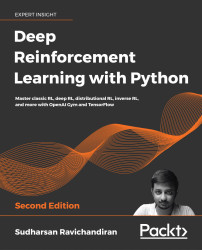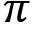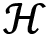•#### Deep Reinforcement Learning with Python - Second Edition#### Overview of this book

With significant enhancements in the quality and quantity of algorithms in recent years, this second edition of Hands-On Reinforcement Learning with Python has been revamped into an example-rich guide to learning state-of-the-art reinforcement learning (RL) and deep RL algorithms with TensorFlow 2 and the OpenAI Gym toolkit. In addition to exploring RL basics and foundational concepts such as Bellman equation, Markov decision processes, and dynamic programming algorithms, this second edition dives deep into the full spectrum of value-based, policy-based, and actor-critic RL methods. It explores state-of-the-art algorithms such as DQN, TRPO, PPO and ACKTR, DDPG, TD3, and SAC in depth, demystifying the underlying math and demonstrating implementations through simple code examples. The book has several new chapters dedicated to new RL techniques, including distributional RL, imitation learning, inverse RL, and meta RL. You will learn to leverage stable baselines, an improvement of OpenAI’s baseline library, to effortlessly implement popular RL algorithms. The book concludes with an overview of promising approaches such as meta-learning and imagination augmented agents in research. By the end, you will become skilled in effectively employing RL and deep RL in your real-world projects.
PrefaceFundamentals of Reinforcement LearningFree Chapter
A Guide to the Gym ToolkitThe Bellman Equation and Dynamic ProgrammingMonte Carlo MethodsUnderstanding Temporal Difference LearningCase Study – The MAB ProblemDeep Learning FoundationsA Primer on TensorFlowDeep Q Network and Its VariantsActor-Critic Methods – A2C and A3CLearning DDPG, TD3, and SACTRPO, PPO, and ACKTR MethodsDistributional Reinforcement LearningImitation Learning and Inverse RLDeep Reinforcement Learning with Stable BaselinesReinforcement Learning FrontiersOther Books You May EnjoyIndexAppendix 1 – Reinforcement Learning Algorithms# Soft actor-critic

Now, we will look into another interesting actor-critic algorithm, called SAC. This is an off-policy algorithm and it borrows several features from the TD3 algorithm. But unlike TD3, it uses a stochastic policy. SAC is based on the concept of entropy. So first, let's understand what is meant by entropy. Entropy is a measure of the randomness of a variable. It basically tells us the uncertainty or unpredictability of the random variable and is denoted by.

If the random variable always gives the same value every time, then we can say that its entropy is low because there is no randomness. But if the random variable gives different values, then we can say that its entropy is high.

For an example, consider a dice throw experiment. Every time a dice is thrown, if we get a different number, then we can say that the entropy is high because we are getting a different number every time and there is high uncertainty since we don't know which number will...